## 1 Introduction

Nash equilibrium is the most important concept in noncooperative game theory.

When dynamic games are considered, researchers usually examine two types of Nash equilibria—open loop Nash equilibria and closed loop Nash equilibria. These classes of equilibria are usually not equivalent in any sense, unlike closed and open loop solutions of deterministic optimal control problems, from which dynamic games originate. Moreover, in some dynamic games, including most of zero-sum games, there is no open loop Nash equilibrium, while a closed loop Nash equilibrium usually exists. The simplest and most illustrative examples are pursuit–evasion games around a pond.

In this paper, an equivalence theorem between closed loop and open loop Nash equilibria is proven for a very large class of deterministic dynamic games with a continuum of players and discrete time.

With this result, we can use the Bellman equation to calculate open loop Nash equilibria, as well as Lagrange multipliers techniques to calculate closed loop Nash equilibria, or to combine both methods. Applying each of these three procedures to games in which such an equivalence result does not hold may lead to erroneous results.

Non-equivalence between closed loop and open loop Nash equilibria is a well known fact in dynamic games. Beside papers concentrating on only one kind of equilibrium, there are numerous papers, especially with economic applications, in which both kinds of Nash equilibria are calculated and compared (see e.g. Fersthman, Kamien  or Cellini, Lambertini ).

The literature focused on equivalence is rather scarce.

The first group consists of papers stating special cases, when an open loop equilibrium is also a closed loop equilibrium: Clemhout, Wan , Fersthman , Feichtinger , Leitmann, Schmittendorf , Cellini, Lambertini, Leitmann , Dragone, Cellini, Palestini  and Reinganum . This equivalence means that an open loop equilibrium is in fact a degenerate feedback equilibrium, which is different from the equivalence result stated in this paper.

There is also a paper by Fudenberg, Levine  examining two stage games with a continuum of players, but without state variables at all. Because of lack of state variables, the authors present an example of a game with a continuum of players in which the equivalence between closed loop and open loop Nash equilibria does not hold. To obtain this, they assume that players are able to observe the past actions of all the continuum of players, which is against the assumptions that gave birth to games with a continuum of players.

A separate introduction to games with a continuum of players can be found at the end of this section.

There are also papers by Wiszniewska-Matyszkiel concerning dynamic games with a continuum of players without private state variables, but with some global state variable only: . The author proves various equivalence results between a dynamic Nash equilibrium profile and a sequence of equilibria in one stage games along this profile. Those results are referred to as decomposition theorems and two of them are presented in Sect. 3. Those papers are not focused on the problem whether the set of open loop Nash equilibria coincides in some way with the set of closed loop Nash equilibria, so only one kind of dynamic equilibria is considered in each of them.

This paper considers deterministic discrete time dynamic games (or multistage games), as defined in contemporary dynamic game theory (see e.g. Başar and Olsder ), in which there are state variables changing in response to players’ decisions, and with a continuum of players. There are two types of state variables: a global state variable and private state variables of players.

For such games, equivalence between open loop Nash equilibria (i.e. Nash equilibria for a game in which players’ strategies depend on time only) and closed loop Nash equilibria (i.e. Nash equilibria for an analogous game in which players’ strategies depend also on current values of observed state variables) of the same open loop form is proven. This implies that we can mix the techniques used to calculate open loop and closed loop Nash equilibria: the Lagrange multiplier method (or, more precisely, Karush–Kuhn–Tucker necessary conditions) and Bellman equation, respectively.

This class of dynamic games has never been considered in papers concerning possible equivalence between closed loop and open loop Nash equilibria. Filling this gap is very important, since such games have numerous applications in modelling many real life problems, e.g. large financial markets, global ecological problems or some public goods problems. Moreover, the calculation of Nash equilibria in games with a continuum of players is often substantially simpler than in games with only finitely many players, while the results for the case of a continuum of players may be regarded as an approximation of results for finitely many players.

### 1.1 Games with a Continuum of Players

Games with a continuum of players were introduced in order to illustrate situations, where the number of agents is large enough to make a single player insignificant—negligible—when we consider the impact of his/her action on aggregate variables, while joint action of the whole set of such negligible players is not negligible. This happens in many real situations: on competitive markets, stock exchange, or when we consider emission of greenhouse gases and similar global effects of exploitation of the common global ecosystem.

Although it is possible to construct models with countably or even finitely many players to illustrate this negligibility, they are very inconvenient to cope with.

The first attempts to use models with a continuum of players are contained in Aumann [16, 17] and Vind .

Some theoretical works on large games are Schmeidler , Mas-Colell , Balder , Wieczorek [22, 23], Wieczorek and Wiszniewska , Wiszniewska-Matyszkiel . An extensive survey of such games is Khan and Sun .

The general theory of dynamic games with a continuum of players is still being developed. On one hand, there are papers on decomposition theorems by Wiszniewska-Matyszkiel cited in Sect. 3, on the other hand, there is a new branch of stochastic mean-field games represented by e.g. Lasry, Lions , Weintraub, Benkard and Van Roy , Huang, Caines and Malhamé  or Huang, Malhamé, and Caines .

There are already many interesting applications of dynamic games with a continuum of players. Some examples are Wiszniewska-Matyszkiel [31, 32] concerning models of exploitation of common ecosystems by large groups of players, Karatzas, Shubik and Sudderth  and Wiszniewska-Matyszkiel [13, 34] analyzing dynamic games with a continuum of players modeling financial markets, Miao  modeling competitive equilibria in economies with aggregate shocks, Wiszniewska-Matyszkiel  and Huang  analyzing an oligopolistic market treated as a mixed large game (with both atomic players and a continuum part), and  containing an example of a dynamic game modeling presidential elections preceded by a campaign. Other models of election using games with a continuum of players are in Ekes .

Introducing a continuum of players instead of a finite number—however, large—can essentially change properties of equilibria and simplify their calculation, even if the measure of the space of players is preserved in order to make the results comparable. At the same time, equilibria in games with a continuum of players constitute a kind of limit of the corresponding equilibria in games with finitely many players, and, sometimes, this limit can be reached even in finitely many steps, which makes the results obtained in this paper directly applicable to games with finitely many players. Such comparisons were made by the author in [39, 40]. Moreover, in , the limit is attained already for finitely many players.

## 2 Formulation of the Problem

Generally, the definition of a noncooperative game in its strategic (or normal) form requires stating its set of players, sets of players’ strategies (and, consequently, strategy profiles) and payoff functions of the players. In dynamic games, especially dynamic games with a continuum of players, those terms require introduction of some primary components.

We consider a dynamic game with a continuum of players—the set of players being the unit interval $$\Bbb{I}$$ with Lebesgue measure λ (and the σ-field of Lebesgue measurable sets denoted by $$\frak{L}$$). Players are represented by points of $$\Bbb{I}$$.

If, instead of points, we considered players as non-negligible and mutually exclusive subsets with union $$\Bbb{I}$$, which can be understood as a partition into coalitions of players, then, even if players from the same subset were identical and chose the same strategy, the results would differ substantially, which is illustrated by Example 6.2.

The time set $$\Bbb{T}$$ is discrete—without any loss of generality $$\Bbb{T}=\{0,\ldots,T\}$$ or $$\Bbb{T}=\{0,1,\ldots\}$$ (we refer to the latter case as time horizon T=+∞). We also use the auxiliary notation $$\overline{\Bbb{T}}$$ to denote the set {0,…,T+1} if the time horizon T is finite, while in the opposite case $$\overline{ \Bbb {T}}=\Bbb{T}$$ (it stands for the time set extended by the time instant after termination of the game, if possible).

Every player i has his/her own private state variable $$w_{i}\in \Bbb{W}_{i}\subset\Bbb{W}$$, whose trajectory is denoted by $$W_{i}: \overline{\Bbb{T}}\rightarrow\Bbb{W}$$. There is also a σ-field $$\mathcal{W}$$ of subsets of $$\Bbb{W}$$. Obviously, $$\Bbb{W}_{i}\in \mathcal{W}$$.

There is also a global (or external) state variable $$x\in\Bbb{X}$$ with trajectory denoted by $$X:\overline{\Bbb {T}}\rightarrow \Bbb{X}$$ and a σ-field $$\mathcal{X}$$ of subsets of $$\Bbb{X}$$.

The set of actions (or decisions) of player i is $$\Bbb {D}_{i}\subset\Bbb{D}$$, but there are also constraints defined by the correspondence of available actions $$D_{i}:\Bbb{T}\times\Bbb{W}_{i}\times\Bbb{X\rightrightarrows D}_{i}$$. We also need a σ-field $$\frak{D}$$ of subsets of $$\Bbb{D}$$. Obviously, $$\Bbb{D}_{i}\in\frak{D}$$.

Any $$\frak{L} -\frak{D}$$-measurable function $$\delta:\Bbb {I}\rightarrow\Bbb{D}$$, such that δ(i)∈D i (t,w i ,x) for $$w=\{w_{i}\}_{i\in\Bbb{I}}\in\Bbb{W}^{\Bbb{I}}$$ and $$x\in\Bbb {X}$$, is called a static profile (of players’ strategies) available at w and x. The set of all the static profiles (of players strategies) available at w and x is denoted by $$\mathfrak{SP} (t,w,x)$$, while the union of these sets—by $$\mathfrak{SP}$$.

Generally, by the term profile of players’ strategies or, for short, profile, we understand an assignment of strategies to the players. In the literature on n-player games, the term n-tuple of strategies is often used instead.

In this paper, we consider three types of profiles.

First come static profiles, which we have just defined. They represent functions assigning strategies to players in one stage games of which the original dynamic game consists. Static profile is not only an auxiliary concept to define dynamic game. As it is shown in Sect. 3, static profiles appear naturally in decomposition theorems, which simplify the calculation of equilibria in a dynamic game (Theorem 3.3).

In the original dynamic game, we consider open loop profiles, in which players’ strategies depend only on time, and closed loop profiles, in which strategies depend also on current values of state variables.

The influence of a static profile on the global state variable is via its m-dimensional statistic, where m is any fixed positive integer.

The statistic function $$U:\mathfrak{SP} \rightarrow\Bbb{R}^{m}$$ is defined by $$U(\delta):= [ \int_{\Bbb{I}}g_{k}(i,\delta (i))d\lambda(i) ] _{k=1}^{m}$$ for a collection of functions $$g_{k}:\Bbb{I}\times\Bbb{D}\rightarrow\Bbb{R}$$ which are $$\frak{L} \otimes\frak{D}$$-measurable for every k.

In most applications and theoretical papers on games with a continuum of players, the statistic is one dimensional and it is usually the aggregate of players’ decisions, which corresponds to g(i,d)=d. If some higher dimensional statistic is used, it may encompass also higher moments of static profiles or the aggregates over several non-negligible subsets of players.

We assume that the statistic is always well defined (which holds e.g. if the functions g k are integrably bounded and $$\Bbb{D}_{i}$$ are bounded). The set of all profile statistics is denoted by $$\Bbb{U}$$.

In order to define payoff functions of players, we first introduce an instantaneous payoff function of player i, $$P_{i}:\Bbb {D}\times\Bbb{U} \times\Bbb{W}\times\Bbb{X}\times\Bbb{T\rightarrow }\Bbb{R}\cup\{-\infty\}$$. Given state variables w i and x and time t, if the players choose a static profile δ, then player i obtains instantaneous payoff P i (δ(i),U(δ),w i ,x,t), i.e., instantaneous payoffs depend on player’s own strategy at the profile, the statistic of the profile, private state variable of the player, global state variable and some dependence on time excluding discounting (e.g. seasonality).

When the time horizon is finite, players obtain also terminal payoffs $$G_{i}:\Bbb{W} \times\Bbb{X}\rightarrow\Bbb{R}\cup\{-\infty\}$$ after termination of the game (for uniformity of notation we include them also in the case of the infinite horizon by assuming that G i ≡0).

At the beginning of the game we have initial conditions $$X(0)=\bar{x}$$ and $$W_{i}(0)=\bar{w}_{i}$$ for $$i\in\Bbb{I}$$.

The regeneration functions of the state variables are $$\phi:\Bbb {X}\times \Bbb{U}\rightarrow\Bbb{X}$$ for the global state trajectory X and $$\kappa _{i}:\Bbb{W}\times\Bbb{X}\times\Bbb{D}\times\Bbb{U}\rightarrow\Bbb {W}_{i}$$ for the private state trajectories W i , and they define these trajectories as follows. If at a time instant t a static profile δ is chosen, then

$$X(t+1)=\phi\bigl(X(t),U(\delta)\bigr)$$

and

$$W_{i}(t+1)=\kappa_{i}\bigl(W_{i}(t),X(t),\delta(i),U(\delta)\bigr).$$

Now it is time to introduce the notion of dynamic strategies and dynamic profiles.

First, we need some auxiliary notation.

If $$\Delta:\Bbb{T}\rightarrow\mathfrak{SP}$$ represents choices of static profiles at various time instants, then we denote by U(Δ) the function $$u:\Bbb{T}\rightarrow\Bbb{U}$$ such that u(t)=U(Δ(t)). The set of all such functions u is denoted by $$\frak{U}$$.

Given a function $$u\in\frak{U}$$ (representing the statistics of profiles chosen at various time instants), the external system evolves according to the equation X(t+1)=ϕ(X(t),u(t)) with the initial condition $$X(0)=\bar{x}$$.

Such a trajectory of the global state variable is said to be corresponding to u and we denote it by X u.

If u=U(Δ), then, by a slight abuse of notation, we write X Δ instead of X U(Δ).

Given functions $$u:\Bbb{T}\rightarrow\Bbb{U}$$ and $$\omega:\Bbb {T}\rightarrow \Bbb{D}_{i}$$ representing, respectively, the statistic of static profiles chosen at various time instants and decisions of player i at those time instants, the private state variable of player i evolves according to the equation W i (t+1)=κ i (W i (t),ω(t),X u(t),u(t)), with the initial condition $$W_{i}(0)=\bar{w}_{i}$$.

Such a trajectory of the private state variable is said to be corresponding to ω and u and it is denoted by $$W_{i}^{\omega,u}$$.

If a function $$\Delta:\Bbb{T}\rightarrow\mathfrak{SP}$$ represents choices of profiles at various time instants, then the trajectory of private state variables W Δ defined by $$( W^{\Delta} ) _{i}:=W_{i}^{\Delta(\cdot) (i),U(\Delta)}$$ is called corresponding to Δ and denoted by W Δ (with coordinates $$W_{i}^{\Delta}$$).

Now it is time to define open and closed loop strategies and profiles in the dynamic game.

A strategy of a player in game theory is a function assigning an available decision to the information about the current state of the game that the player takes into account. For open loop strategies, the only information that the player takes into account at each stage of the game is time. This definition is identical in all the dynamic games and optimal control literature.

The concept of closed loop is not so unequivocal. This term is used with at least three different meanings. In each of them, the current value of the state variable is a part of information. In this paper, closed loop strategy uses information consisting of both the private state variable of the player and the global state variable, as well as time.

Formally, we have the following definitions.

A function $$\omega:\Bbb{T}\rightarrow\Bbb{D}_{i}$$ is called an open loop strategy of player i. It is called admissible at $$u\in\frak{U}$$ iff for every $$t\in\Bbb{T}$$, $$\omega(t)\in D_{i}(t,W_{i}^{\omega ,u}(t),X^{u}(t))$$. Note that, in general, if we do not know the statistic, we cannot say whether a strategy is admissible—it may be treated only as the player’s plan and its realization depends on the behavior of the state variables, which, in turn, depends on the strategies of the other players.

An open loop profile (of players’ strategies) is any function $$\varOmega:\Bbb{I}\times\Bbb{T}\rightarrow\Bbb{D}$$ such that $$\{\varOmega(i,\cdot)\}_{i\in\Bbb{I}}$$ is a collection of open loop strategies admissible at $$u\in\frak{U}$$ defined by u(t)=U(Ω(⋅,t)) and such that for every t, Ω(⋅,t) is $$\frak {L} -\frak{D}$$-measurable.

Equivalently, we may represent it as $$\Delta:\Bbb{T}\rightarrow \mathfrak{SP}$$ such that for every t, $$\Delta(t)\in\mathfrak{SP} (t,W^{\Delta}(t),X^{\Delta}(t))$$. Therefore, we use analogous notation for the trajectories corresponding to an open loop profile, $$W_{i}^{\varOmega}$$ and X Ω, and for its statistics U(Ω). The set of all the open loop strategies of player i admissible at u is denoted by OL i (u), while the set of all the open loop profiles is denoted by $$\mathcal{OL}$$.

A function $$\psi:\Bbb{T}\times\Bbb{X}\times\Bbb{W}_{i}\rightarrow \Bbb{D}_{i}$$ is called a closed loop strategy of player i iff for every $$t\in \Bbb{T}$$, $$x\in\Bbb{X}$$ and $$w_{i}\in\Bbb{W}_{i}$$, we have ψ(t,x,w i )∈D i (t,w i ,x). Note that, unlike the definition of open loop strategies, we can guarantee admissibility already in the definition of strategy.

A closed loop profile (of players’ strategies) is any function $$\varPsi:\Bbb{I}\times\Bbb{T}\times\Bbb{X}\times\Bbb{W}\rightarrow \Bbb{D}$$ satisfying the following condition: $$\{\varPsi(i,\cdot,\cdot,\cdot)\}_{i\in\Bbb{I}}$$ is a collection of closed loop strategies such that for every $$t\in\Bbb{T}$$ and $$x\in\Bbb{X}$$, the function Ψ(⋅,t,x,⋅) is $$\frak {L} \otimes \mathcal{W}-\frak{D}$$-measurable (which implies measurability of the function iΨ(i,t,x,w i ) for every measurable function iw i ). The set of all the closed loop strategies of player i is denoted by CL i , while the set of all the closed loop profiles is denoted by $$\mathcal{CL}$$.

For a closed loop profile $$\varPsi\in\mathcal{CL}$$, we define its open loop form $$\varPsi^{\mathrm{OL}}\in\mathcal{OL}$$ and the corresponding trajectories of state variables X Ψ and W Ψ, recursively, by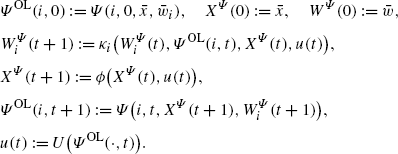For an open loop profile $$\varOmega\in\mathcal{OL}$$, we define its closed loop form $$\varOmega^{\mathrm{CL}}\in\frak{P}(\mathcal{CL})$$ by $$\varOmega^{\mathrm{CL}}:= \{ \varPsi\in\mathcal{CL}:\varPsi(i,t,X^{\varOmega }(t),W_{i}^{\varOmega}(t))=\varOmega(i,t)\text{ for a.e. }i \text{ and every } t \}$$.

The payoff that a player obtains in the game is equal to the suitably discounted sum of instantaneous payoffs obtained during the game and the terminal payoff. It is always a function from the set of profiles into extended reals. For our two types of information structure defining sets of strategies—open and closed loop—we have, respectively, $$\varPi_{i}^{\mathrm{OL}}:\mathcal{OL}\rightarrow\overline{\Bbb{R}}$$ and $$\varPi_{i}^{\mathrm{CL}}:\mathcal{CL}\rightarrow\overline{\Bbb{R}}$$.

For an open loop profile Ω, the payoff of player i is defined by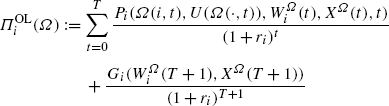for a constant r i >0, called the discount rate of player i.

The definition of a closed loop profile is an obvious consequence—we can simply define it by $$\varPi_{i}^{\mathrm{CL}}(\varPsi):=\varPi_{i}^{\mathrm{OL}}(\varPsi^{\mathrm{OL}})$$.

Without any additional assumptions, payoffs do not have to be well defined in the infinite time horizon, therefore we add the assumption that they are well defined (this is satisfied if, e.g., P i are bounded from above). Then we have well defined $$\varPi_{i}^{\mathrm{OL}}$$ and $$\varPi_{i}^{\mathrm{CL}}$$, which completes the definition of the two kinds of games: with open loop information structure and with closed loop information structure.

### 2.1 Nash Equilibria

One of the basic concepts in game theory, the Nash equilibrium, assumes that every player chooses a strategy which maximizes his/her payoff given the strategies of the remaining players. In the case of games with a continuum of players, the term “every” has to be replaced by “almost every”.

In order to simplify the notation, we need the following abbreviation: for a profile S and a strategy d of player i, the symbol S i,d for a strategy d of player i denotes the profile such that S i,d(i)=d and S i,d(j)=S(j) for ji.

### Definition 2.1

A profile S is a Nash equilibrium iff for a.e. $$i\in\Bbb{I}$$ and every strategy d of player i, we have Π i (S)≥Π i (S i,d).

This definition applies both to open and closed loop Nash equilibria—in each of these cases strategies/profiles are open or closed loop strategies/profiles, respectively, and the appropriate payoff function is applied.

## 3 Games Without Private State Variables—Decomposition and Equivalence

In this section, we recall some existing results concerning games without private state variables and derive their obvious consequence.

For simplicity of notation, we omit the private state variables w.

In this section, we use the notion of static equilibrium at a time t and state x: this is a Nash equilibrium in a one shot game played at the time instant t at state x in which players’ sets of strategies are D i (t,x), while the payoff functions are instantaneous payoffs P i (⋅,⋅,x,t).

It may seem that static equilibria are useless in dynamic games. However, in earlier papers of the author, various decomposition results are proven, stating how an equilibrium in the original dynamic game can be decomposed into a coupled sequence of equilibria in one stage games.

In Wiszniewska-Matyszkiel , a wide class of discrete time dynamic games with a continuum of players and open loop strategies is considered. We can use Theorem 5.1(ii) of that paper in a form updated to the games considered in this paper.

### Theorem 3.1

We consider two conditions.

1. (*)

For every t, the static profile Ω(⋅,t) is a static equilibrium at time t and state X Ω(t);

2. (**)

The open loop profile Ω is an open loop Nash equilibrium.

For every $$\varOmega\in\mathcal{OL}$$, condition (*) implies (**).

If for a.e. player i, the payoff $$\varPi_{i}^{\mathrm{OL}}(\varOmega)$$ is finite, then (*) and (**) are equivalent.

In Wiszniewska-Matyszkiel , an analogous theorem for games with arbitrary time set is proven. We do not cite it here, since—in order to make it work in a more general environment—the assumptions necessary to obtain equivalence are stronger (similar to those in Theorem 3.2 below) and, therefore, in the case of discrete time, that result is weaker than Theorem 3.1.

In Wiszniewska-Matyszkiel , a wide class of stochastic games with a continuum of players and closed loop strategies are considered. We can use Theorem 1 of that paper (to be more specific: b and f of it) simplified to suit the deterministic games considered in this paper.

### Theorem 3.2

1. (a)

If Ψ is a closed loop profile and, for all t, the static profiles Ψ(⋅,t,X Ψ(t)) are static equilibria at time t and state of the system X Ψ(t), then Ψ is a closed loop Nash equilibrium.

2. (b)

Let the space of strategies $$\Bbb{D}$$ be such that $$\operatorname{diag}\Bbb {D}:=\{(d,d):d \in\Bbb{D}\}$$ is $$\mathcal{D}\otimes\mathcal{D}$$-measurable and $$\Bbb{D}$$ is a measurable image of a measurable space $$(\Bbb{Z},\mathcal{Z})$$ that is an analytic subspace of a separable compact topological space $$\Bbb{S}$$ (with the σ-field of Borel subsets $$\mathcal{B}(\Bbb{S})$$). Assume that, for a.e. i, t and every u, x, the function P i (⋅,u,x,t) is upper semi-continuous, for a.e. i, the function P i is such that inverse images of measurable sets are $$\mathcal{D}\otimes\mathcal {B}(\Bbb{U})\otimes\mathcal{X}\otimes\mathcal{P}(\Bbb{T})$$-analytic and the correspondence D i has an $$\mathcal{X}\otimes\mathcal{D}$$-analytic graph and compact values. Every closed loop Nash equilibrium Ψ such that, for almost every player i, the payoff $$\varPi_{i}^{\mathrm{CL}}(\varPsi (i,\cdot,\cdot),U(\varPsi),X^{\varPsi})$$ is finite, satisfies the following condition: for all t, static profiles Ψ(⋅,t,X Ψ(t)) are static equilibria at time t and state of the system X Ψ(t).

The assumptions are satisfied in quite a general framework: e.g. measurability of the diagonal in the product σ-field holds for every complete separable metric space (or, even more generally, for its continuous image) with the σ-field of Borel subsets, and, usually, if we cope with sets which are not measurable, then they are projections or continuous images of measurable sets, which are usually analytic. Nevertheless, even these assumptions, especially continuity and compactness assumptions, are too restrictive and can be weakened, as we do in Theorem 3.3, in which we assemble and generalize results stated in Theorems 3.1 and 3.2.

### Theorem 3.3

1. (a)

If Ψ is a closed loop profile and, for all t and x, the static profiles Ψ(⋅,t,x) are static equilibria at time t and state of the system x, then Ψ is a closed loop Nash equilibrium.

2. (b)

If Ψ is a closed loop profile and, for every t, the static profiles Ψ OL(⋅,t) are static equilibria at time t and state of the system X Ψ(t), then Ψ is a closed loop Nash equilibrium.

3. (c)

If Ω is an open loop profile and, for every t, the static profiles Ω(⋅,t) are static equilibria at time t and state of the system X Ω(t), then Ω is an open loop Nash equilibrium.

4. (d)

Every closed loop Nash equilibrium Ψ such that for almost every player i, the payoff $$\varPi_{i}^{\mathrm{CL}}(\varPsi(i,\cdot,\cdot),U(\varPsi ),X^{\varPsi})$$ is finite, satisfies the following condition: for all t, static profiles Ψ(⋅,t,X Ψ(t)) are static equilibria at time t and state of the system X Ψ(t).

5. (e)

Every open loop Nash equilibrium Ω such that for almost every player i, the payoff $$\varPi_{i}^{\mathrm{OL}}(\varOmega(i,\cdot),U(\varOmega ),X^{\varOmega})$$ is finite, satisfies the following condition: for all t, static profiles Ω(⋅,t) are static equilibria at time t and state of the system X Ω(t).

### Proof

Since this theorem contains some parts of Theorem 3.1 and 3.2, the only thing that remains to be proven is d).

Let us take any closed loop Nash equilibrium Ψ with finite payoff of almost every player and any t such that Ψ(t,X Ψ(t)), which, for brevity, we denote as δ, is not a static Nash equilibrium. It means that there exists a set $$\Bbb {J}\subset\Bbb{I}$$ of positive measure for which, for every $$i\in\Bbb{J}$$, there exists dD i (t,X Ψ(t)) such that we increase instantaneous payoff by changing player’s i strategy to d, since, due to nonatomicity of the measure on the space of players, the statistic does not change.

So, P i (δ(i),U(δ),X Ψ(t),t)<P i (d,U(δ),X Ψ(t),t).

If we change the strategy of player i by only replacing the value of Ψ(i,t,X Ψ(t)) by d, then we change neither the statistic nor the global state trajectory. Therefore, we can increase the instantaneous payoff at time t without changing payoffs in any other time instant, which contradicts the optimality of player’s i strategy. □

As a consequence of Theorem 3.3, we can derive an equivalence result between open and closed loop Nash equilibria. However, we do it in a more general framework.

## 4 Games with Private State Variables—Equivalence

In this section we formulate the main result—equivalence between open loop and closed loop Nash equilibria in a more general case, when there is a nontrivial dependence on private state variables.

### Theorem 4.1

1. (a)

A closed loop profile Ψ is a closed loop Nash equilibrium if and only if Ψ OL is an open loop Nash equilibrium.

2. (b)

An open loop profile Ω is an open loop Nash equilibrium if and only if there exits a profile ΨΩ CL that is a closed loop Nash equilibrium.

3. (c)

An open loop profile Ω is an open loop Nash equilibrium if and only if every profile ΨΩ CL is a closed loop Nash equilibrium.

### Proof

The first thing that should be emphasized is the fact that X is identical for all the profiles with the same open loop form.

Besides, by nonatomicity of λ, changing a strategy by a single player changes neither X nor u.

Therefore, we have independent dynamic optimization problems with X and u treated as parameters only, since player i has negligible influence on them (unlike in games with finitely many players).

The only variable treated as a state variable in the optimization problem of player i remains his/her private state variable w i . Coupling by u does not change anything in players’ optimization given u, since u is identical for every profile with the same open loop form. As is well known, in deterministic problems with perfect information about the state variable, optimization over the sets of closed and open loop strategies leads to equivalent results. □

## 5 Implications for the Calculation of Equilibria

As a consequence of Theorem 3.3, in the case when there is no nontrivial dependence on private state variables, the only procedure we need is the calculation of a sequence of equilibria in static games and construction of a dynamic open/closed loop profile consisting of them. In this case, the problem of finding an equilibrium in a dynamic game is decomposed into static problems coupled by the statistic and the global state variable trajectory.

In the nontrivial case, we can find—as in usual dynamic optimization—an open or closed loop equilibrium profile using both methods: the Bellman equation and the Lagrange or Karush–Kuhn–Tucker multiplier method.

If we use a system of discrete time Bellman equations, which generally leads to closed loop equilibria, we can turn it into an open loop equilibrium just by taking the open loop form of it. By Theorem 4.1, it is an open loop equilibrium.

If we use the system of equations from Karush–Kuhn–Tucker necessary conditions, which returns an open loop equilibrium, we can easily turn it into a closed loop equilibrium by taking any representative of its closed loop form.

### 5.1 Discrete Time Bellman Equation System

We start by considering the optimization problem of player i, given the choices of the remaining players, and consequently, the function of statistics over time $$u\in\frak{U}$$ (with the trajectory of the global state variable X u).

If a function $$V_{i}^{u}:\overline{\Bbb{T}}\times\Bbb{W} \rightarrow \overline{\Bbb{R}}$$ represents the value function of this problem, the Bellman equation is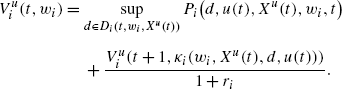The Bellman equation is always considered together with a certain terminal condition.

In the finite horizon case, it is always $$V_{i}^{u}(T+1,w_{i})=G_{i}(w_{i},X^{u}(T+1))$$.

In the infinite horizon case, the terminal condition can have various forms. The simplest of them, which, together with the Bellman equation, is a sufficient condition for payoff optimization by player i, is $$\lim_{t\rightarrow\infty}V_{i}^{u}(t,W_{i}^{\omega,u}(t))\cdot(1+r)^{-t}=0$$ for every open loop strategy ω of player i admissible at u.

If there exists a function $$V_{i}^{u}$$ satisfying the Bellman equation together with an appropriate terminal condition, then it defines the best payoff that can be obtained by player i if the statistics of static profiles chosen over time constitute the function u.

In such a case, every optimal closed loop strategy of player i can be found by solving the inclusion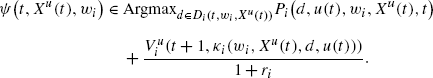Therefore, a closed loop equilibrium profile Ψ can be found by solving the set of inclusions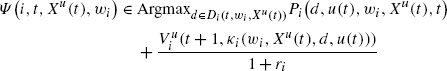for a.e. $$i\in\Bbb{I}$$, with the coupling condition u=U(Ψ OL).

Various versions of Bellman equation, together with appropriate terminal conditions, can be found in e.g. Bellman , Blackwell , Stokey and Lucas  or Wiszniewska-Matyszkiel .

### 5.2 Karush–Kuhn–Tucker Necessary Conditions

Similarly to the previous procedure, if we want to calculate an open loop Nash equilibrium, we first solve the optimization problem of player i, given choices of the remaining players resulting in $$u\in \frak{U}$$.

Player i faces the maximization problem of

$$\sum_{t=0}^{T}\frac{P_{i}(\omega(t),u(t),W_{i}^{\omega ,u}(t),X^{u}(t),t)}{(1+r)^{t}}+ \frac{G_{i}(W_{i}^{\omega ,u}(T+1),X^{u}(T+1))}{(1+r)^{T+1}}$$

over the set of open loop strategies ω admissible at u.

To formulate the necessary conditions, we assume that both functions κ i and P i are continuously differentiable with respect to player’s own strategy and private state variable.

Obviously, the necessary conditions for the optimization of player i depend on the form of the sets of available actions D i (t,w i ,x).

In the simplest case, when these sets are open, or the optimal strategy is always an interior point, we can write Karush–Kuhn–Tucker necessary conditions using the discrete time Hamiltonian $$H_{i}^{u}:\Bbb {D}\times\Bbb{W} \times\Bbb{T}\times\Bbb{R}\rightarrow\Bbb{R}$$. With the costate variable of player i denoted by $$\mu_{i}\in\Bbb{R}$$, it has the form

$$H_{i}^{u}(d,w_{i},t,\mu_{i}):=P_{i} \bigl(d,u(t),w_{i},X^{u}(t),t\bigr)\cdot (1+r)^{-t}+ \mu_{i}\cdot\kappa_{i}\bigl(w_{i},X^{u}(t),d,u(t) \bigr).$$

In this case, the necessary conditions for an open loop strategy ω optimizing the payoff of player i, given u, are for every t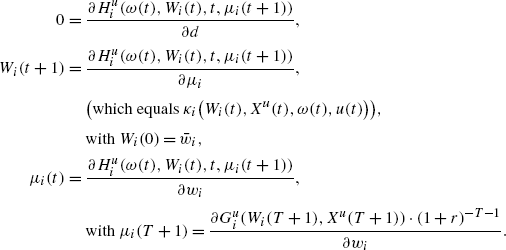For a dynamic open loop profile which is an open loop Nash equilibrium, these necessary conditions hold for almost every player.

As in the case of Bellman equation, the players’ equation system is coupled by u=U(Ω).

This form of necessary conditions can be easily derived from the standard Lagrange multipliers technique.

However, the sets D i (t,w i ,x) are usually not open and we cannot assume that, at every time instant t, ω i (t) is an interior point of D i (t,W i (t),X(t)). In such a case, depending on the form of D i , additional multipliers appear, corresponding to constraints active at this point.

In the case when we have an open set $$\Bbb{D}$$ and $$D_{i}(t,w_{i},x)=\{d\in\Bbb{D}:h(d,w_{i},x)\geq0\}$$, for a differentiable function h with nonzero gradient, the Hamiltonian is replaced by a Hamiltonian with constraints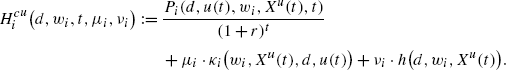The necessary condition contains all the conditions as before, with H replaced by H c and, additionally, ν i (t)≥0, and a complementary slackness condition ν i (t)=0 whenever h(ω)(t,W i (t),X u(t))>0.

If there are more inequality constraints and the constraints satisfy some constraint qualification, e.g. gradients of active constraints are linearly independent at each point of D i (t,W i (t),X u(t)), then we have similar necessary conditions, but we have to consider more multipliers.

Although the formulation of Karush–Kuhn–Tucker necessary conditions using Hamiltonian or Hamiltonian with constraints is not a standard formulation in nonlinear optimization, it seems quite common in dynamic optimization (see e.g. Başar, Older ), and they can be easily derived from the standard textbook form of Karush–Kuhn–Tucker necessary conditions (e.g. like in Bazaraa, Sherali and Shetty ).

## 6 Examples

### 6.1 Common Ecosystem Without Private State Variables

We consider a simple model of a common renewable resource exploited by many users for whom it is the only source of income.

### Example 6.1

The statistic function is the aggregate i.e. g(i,d):=d.

The function describing the behavior of the external ecosystem is ϕ(x,u):=(1+ζ)xu, for ζ>0, called the regeneration rate, and the initial state is $$\bar{x}>0$$.

Since the ecosystem is a common property, the private states do not change and they are skipped for simplicity of notation.

The set of available strategies is D i (t,x):=[0,cx], where c is a constant satisfying c≤1+ζ (which guarantees that the players are not able to extract more than is available).

The instantaneous payoff functions are P i (d,u,x,t):=lnd, with ln0 understood as −∞. The discount rate is r>0, identical for all the players.

The time horizon is either a finite T or +∞.

In the case of finite T, the terminal payoff is G i (x):=lnx.

In this example, the so called “tragedy of the commons” is present in a very drastic form. In the case of a continuum of players, considered in this paper, the equilibrium extraction of the players can be high enough to lead to total destruction of the resource in finite time and, consequently, players’ payoffs are equal to −∞, if possible.

### Proposition 6.1

1. (a)

If c=1+ζ, then no open/closed loop dynamic profile such that a set of players of positive measure get finite payoffs is an equilibrium, and every dynamic profile yielding the destruction of the system in finite time (i.e. there exists $$\bar{t}$$ such that $$\forall t>\bar{t}$$, X(t)=0) is an open/closed loop Nash equilibrium. For every open/closed loop Nash equilibrium, for a.e. player, the payoff is −∞.

2. (b)

If c<1+ζ, then the only (up to measure equivalence of the open loop forms) closed loop Nash equilibria are profiles Ψ such that Ψ OL(i,t)=cX Ψ(t) for all i, t.

3. (c)

If c<1+ζ, then the only (up to measure equivalence) open loop Nash equilibrium is the profile Ω such that $$\varOmega(i,t)=c\cdot\bar {x} \cdot ( 1+\zeta-c ) ^{t}$$ (the profile defined by the equation Ω(i,t)=cX Ω(t)).

### Proof

By the decomposition Theorem 3.3, every open or closed loop Nash equilibrium of finite payoff is composed of static Nash equilibria.

The only static Nash equilibria are the static profiles at which almost every player extracts the maximal amount cx.

In the case when c<1+ζ, every profile is such that the trajectory of the state variable X(t) is bounded from below by $$\bar{x} \cdot ( 1+\zeta-c ) ^{t}$$ and from above by $$\bar{x} \cdot( 1+\zeta) ^{t}$$. This gives a finite upper bound for player’s payoff and a finite lower bound for the player’s optimal payoff, given the behavior of the remaining players resulting in the global state variable trajectory X.

Therefore, a profile in which a set of players of positive measure gets payoff −∞ cannot be a Nash equilibrium and every Nash equilibrium has finite payoff. This completes the proof of (b) and (c).

In (a), if there existed a profile with finite payoffs for almost every player, then it would consist of static Nash equilibria, and, consequently, almost every player would extract c=1+ζ. This would result in X(1)=0 and payoff equal to −∞ for almost every player—a contradiction. □

### 6.2 An Analogue with Finitely Many Players

For comparison, we present a simple example showing what happens if instead of a continuum we consider finitely many players.

For maximal simplicity of calculations, we consider a two stage game (i.e. T=1). We consider a modification of Example 6.1 with n players.

We can think of it as of the initial game in which the continuum of players is divided into n identical subsets, players in one subset choosing identical decision in order to maximize the payoff of the whole subset, treated as one decision maker.

Such an approach often appears in economics, especially in macroeconomics, and it is called the representative consumer approach. In cooperative game theory such sets are called coalitions. A measure $$\frac{1}{n}$$ is assigned to each of such artificial players. Therefore, the aggregates are preserved and the statistic of a profile remains the same.

### Example 6.2

This example differs from Example 6.1 only by the measure space of players: the set of players is {1,…,n} with a measure (by a slight abuse of notation let us denote it also by λ) being the normed counting measure, i.e. $$\lambda(i):=\frac{1}{n}$$.

We consider only the case of c=1+ζ and T=1.

All the other objects remain the same (we do not even have to change notation in the definition of the statistic, since we can understand the integral as the Stieltjes integral with respect to the new λ).

So, as before, we have an open loop Nash equilibrium problem and a closed loop Nash equilibrium problem—the only difference in their definitions is that the statistic is the mean of n strategies (instead of the Lebesgue integral over the unit interval).

Nevertheless, it should be noted that each player has a non-negligible impact on u and, consequently, on X u. Therefore, when using Bellman equation or Karush–Kuhn–Tucker conditions, as described in Sect. 5, for the optimization problem of player i, we have to treat X as his/her private state variable and do not lose influence of his/her own strategy on the statistic.

We are only interested in symmetric equilibria.

First, we consider the game with open loop strategies.

In order to find a symmetric open loop Nash equilibrium, we first look for the best response of player i to a profile Ω in which the other players choose Ω(i,t)=b t for t=0,1.

The optimization problem of player i in this case is to find an open loop strategy ω maximizing

$$\ln\omega(0)+ \frac{1}{1+r} \ln\omega(1) +\frac{1}{(1+r)^2} \ln X(2),$$

with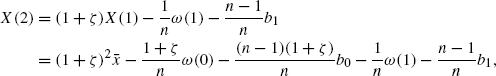subject to the constraints 0≤ω(0)≤(1+ζ)X(0) and 0≤ω(1)≤(1+ζ)X(1).

After calculation of the best response, we substitute ω(i)=b i and we get the unique symmetric open loop equilibrium:

$$\omega(0)=\frac{n(1+\zeta) \bar{x}(1+r)^2}{1+n(1+r)+n(1+r)^2}=\varOmega(i,0)$$

and

$$\omega(1)= \frac{n(1+\zeta)^2 \bar{x}(1+r)}{1+n(1+r)+n(1+r)^2}=\varOmega(i,1),$$

with all constraints satisfied with strict inequalities.

In order to find a closed loop Nash equilibrium, we use the Bellman equation. Again, we assume that the remaining n−1 players choose identical closed loop strategies Ψ(i,t,x)=b t (x), we find ψ(t,x) as the best response of player i to them. Afterwards, we substitute b t (x)=ψ(t,x). The value function of player i, V i , is calculated recursively, starting with time 2.

V i (2,x)=lnx.

Using this, we can calculate

$$V_i(1,x)=\max_{d\in[0,cx]}\ln d +\frac{1}{1+r}V\biggl(2,(1+\zeta)x-\frac {1}{n}d-\frac{n-1}{n}b_1(x)\biggr),$$

which gives $$V_{i}(1,x)=\frac{2+r}{1+r} \ln x+ \text{const}$$, necessary to find the optimum at time 0, the optimum at time 1 is attained at

$$d=\min\biggl(\frac{(1+r)[n(1+\zeta)x-(n-1)b_1(x)]}{2+r},(1+\zeta)x\biggr),$$

and, after substituting d=b 1(x), we get

$$\psi(1,x)=\frac{(1+r)n(1+\zeta)x}{1+n(1+r)}=\varPsi(i,1,x).$$

And, finally,

$$V_i(0,x)=\max_{d\in[0,cx]}\ln d +\frac{1}{1+r}V\biggl(1,(1+\zeta)x-\frac {1}{n}d-\frac{n-1}{n}b_0(x)\biggr),$$

the optimum is attained at

$$d=\min\biggl(\frac{(1+r)^2[n(1+\zeta)x-(n-1)b_0(x)]}{2+r+(1+r)^2},(1+\zeta)x\biggr),$$

and, after substituting d=b 0(x), we get

$$\psi(0,x)=\frac{(1+r)^2 n(1+\zeta)x}{2+r+n(1+r)^2}=\varPsi(i,0,x).$$

As we can see that, for any finite n, these two kinds of equilibria are not equivalent in any sense.

We can also see that, as n tends to infinity, the closed loop Nash equilibria in this example converge to a closed loop Nash equilibrium of Example 6.1, while there is no such convergence for open loop Nash equilibria.

### 6.3 Common Ecosystem with Private State Variables

In this example, we reformulate Example 6.1 in another direction, in order to take into account the fact that instantaneous payoffs can be partly invested to build a kind of capital which increases effectiveness of exploitation. This is described by a one dimensional private state variable w i .

### Example 6.3

The set of players is, as in Example 6.1, the unit interval with Lebesgue measure.

The set of players’ strategies is two-dimensional, since the amount of extracted resource is divided in order to be used in two ways: consumption or investment. So, we have decisions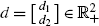with d 1 denoting consumption and d 2 – investment.

The availability condition becomes $$D_{i}(t,w_{i},x)= \{ d\in\Bbb{R}_{+}^{2}:d_{1}+d_{2}\leq w_{i}\cdot x \}$$, where the private state variables, w i ≥0, have some initial conditions defined by a measurable function $$i\mapsto\bar{w}_{i}>0$$.

The statistic function is the aggregate of the exploitation, i.e. the sum of both coordinates of strategy g(i,d):=d 1+d 2.

The utility of consumption remains logarithmic, which partly defines the instantaneous payoff functions. However, since players can increase their constraint on exploitation, it cannot be guaranteed that they do not want to extract more than is available, i.e. that u≤(1+ζ)⋅x. In such a case, the extractions have to be proportionally reduced to the highest admissible level, which leads to the instantaneous payoff function

$$P_{i}(d,u,x,t):=\left \{ \begin{array}{l@{\quad}l} \ln d_{1} & \text{if }u\leq(1+\zeta)\cdot x, \\ \ln\frac{d_{1}(\cdot1+\zeta)\cdot x}{u} & \text{otherwise} \end{array} \right .$$

(again with ln0 understood as −∞).

The function determining the behavior of the global state variable is

$$\phi(x,u):=\left \{ \begin{array}{l@{\quad}l} (1+\zeta)x- u & \text{if }u\leq(1+\zeta)\cdot x, \\ 0 & \text{otherwise,} \end{array} \right .$$

while for the private state variables we have

$$\kappa_{i}(w_{i},x,d,u):=\left \{ \begin{array}{l@{\quad}l} w_{i}+e\cdot d_{2} & \text{if }u\leq(1+\zeta)\cdot x, \\ w_{i}+e\cdot\frac{d_{2}(\cdot1+\xi)\cdot x}{u} & \text{otherwise.} \end{array} \right .$$

We consider both infinite and finite time horizons with G(x):=lnx.

### Proposition 6.2

1. (a)

If $$\int_{\Bbb{I}}\bar{w}_{i}d\lambda(w_{i})\geq 1+\xi$$, then no open/closed loop dynamic profile such that a set of players of positive measure get finite payoffs is an equilibrium, and every dynamic profile yielding the destruction of the system in finite time (i.e. such that $$\exists\bar{t}\leq T\ \forall t>\bar{t}\ X(t)=0$$) is an open/closed loop Nash equilibrium. For every open/closed loop Nash equilibrium, for a.e. player, the payoff is −∞.

2. (b)

Let us consider an open/closed loop Nash equilibrium with finite payoffs. For its open loop form Ω, for a.e. i and every t, we have $$( \varOmega(i,t) ) _{1}+ ( \varOmega(i,t) ) _{2}=W_{i}^{\varOmega }(t)\cdot X^{\varOmega}(t)$$.

3. (c)

Every open/closed loop profile satisfying X(t)=0 for some $$t\in \overline{\Bbb{T}}$$, is an open/closed loop Nash equilibrium.

### Proof

(a) and (b). Assume that there exists an open loop Nash equilibrium profile Ω such that the set of players with finite payoffs is of positive measure.

This implies that X Ω(t)>0 for every $$t\in\overline{\Bbb{T}}$$.

Let us consider the optimization of player i and his/her strategy at some time instant $$\bar{t}$$.

Either $$\varOmega_{1}(i,\bar{t})+\varOmega_{2}(i,\bar{t})=W_{i}^{\varOmega}(\bar {t})\cdot X^{\varOmega}(\bar{t})$$ is satisfied, or $$\varOmega_{1}(i,\bar{t})+\varOmega_{2}(i,\bar{t})<W_{i}^{\varOmega}(\bar {t})\cdot X^{\varOmega}(\bar{t})$$.

If the latter holds, player i can improve his/her instantaneous payoff at time $$\bar{t}$$ without changing any other instantaneous payoff by replacing $$\varOmega_{1}(i,\bar{t})$$ by $$( W_{i}^{\varOmega}(\bar{t})\cdot X^{\varOmega}(\bar{t})-\varOmega _{2}(i,\bar{t}) )$$.

If such players constitute a set of positive measure, then the profile is not a Nash equilibrium.

Otherwise, $$u(\varOmega)(\bar{t})\geq(1+\zeta)X^{\varOmega}(\bar{t})$$. Therefore, $$X^{\varOmega}(\bar{t}+1)=0$$ and the payoff is −∞.

For closed loop profiles, the proof follows from the previous reasoning and the fact that for a closed loop profile that is a closed loop Nash equilibrium, its open loop form should be an open loop Nash equilibrium.

(c) Assuming the remaining players behave according to this profile, the payoff of every player is −∞, whatever strategy he/she chooses, so he/she cannot improve his/her payoff. □

### Proposition 6.3

Let T be finite. For every u $$\in\frak{U}$$ such that for every tT+1, X u(t)>0, the best response of player i to u has the open loop form ω which is unique, and there exist real constants μ(t) (for t=0,…,T+1), positive constants ν 1(t) and nonnegative constants ν 2(t) (for t=0,…,T), such that the following system of equations is satisfied for t=0,…,T: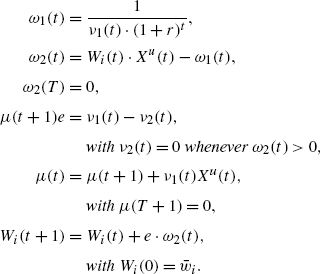### Proof

First, we focus on open loop strategies only.

To find the optimal strategy of player i, we can use an appropriate form of Karush–Kuhn–Tucker conditions.

We have two kinds of inequality constraints which can be active at the optimum:

• ω 1(t)+ω 2(t)≤W i (t)X u(t), which we rewrite as W i (t)X u(t)−ω 1(t)−ω 2(t)≥0 and we assign to this constraint a nonnegative multiplier ν 1(t); and

• ω 2(t)≥0, to which we assign a nonnegative multiplier ν 2(t).

Constraint qualifications hold—the gradients of all the active constraints are linearly independent.

The Hamiltonian with constraints is $${H^{c}_{i}}^{u}(d,w_{i},t,\mu,\nu_{1},\nu_{2}):=\frac{\ln d_{1}}{(1+r)^{t}} +\mu(w_{i} -e d_{2})+\nu_{1} (w_{i} X^{u}(t)-d_{1} -d_{2}) +\nu_{2} d_{2}$$.

The equations are an immediate consequence of Karush–Kuhn–Tucker necessary conditions.

The multipliers ν 1 are strictly positive, since $$\nu_{1}(t)=\frac {1}{\omega_{1}(t)(1+r)^{t}}$$, with ω 1(t) strictly positive.

We also get ω 2(T)=0, since ν 2(T)>0.

Note that the instantaneous payoff is strictly concave, while all the other functions of the model are linear in both player’s strategy and private state variable, so the necessary condition is also sufficient and the maximum is unique.

On the other hand, it exists, since we optimize an upper semi-continuous function over a compact set. □

### Proposition 6.4

Let us consider the case of finite T and the initial $$\bar{w}_{i}$$ identical for all the players.

Every Nash equilibrium profile with open loop form Ω such that X Ω(t)>0 for every tT+1, satisfies the condition for a.e. i, Ω(i,⋅)=ω for the open loop strategy ω defined in Proposition 6.3, given u=ω 1+ω 2.

### Proof

First, let us consider an open loop profile Ω that is an open loop Nash equilibrium.

If $$\bar{w}_{i}$$ are identical for all players, then every player faces the same optimization problem, which has a unique solution, equal to ω defined in Proposition 6.3 for u=U(Ω).

If the profile is a Nash equilibrium, then the payoff of a.e. player is optimal, i.e., in our case, a.e. player chooses a strategy equal to ω from Proposition 6.3. The statistic of a profile at which players’ strategies are identical and equal to ω for a.e. player is equal to ω 1+ω 2.

For closed loop profiles it is immediate by the above reasoning and Theorem 4.1. □

## 7 Conclusions and Further Research

In this paper, equivalence between open loop and closed loop Nash equilibria in a wide class of discrete time dynamic games with a continuum of players has been proven.

Besides the general theory, its implications for the simplification of methods for calculating Nash equilibria of both kinds have been shown.

The results have been used to solve various problems concerning exploitation of a common renewable resource. Besides two examples of such games with a continuum of players, an analogue of one of them with finitely many players has been examined to show substantial differences in results and methods that can be used.

An obvious generalization of the results obtained in this paper can be obtained by extending the notion of a closed loop strategy to a strategy dependent not only on time and current values of state variables, but also on a part of, or even the whole, history of the global variables (the statistic and the global state variable), player’s own past actions and trajectory of his/her private state variable observed at time t. The results proven in this paper remain valid and the proofs follow almost the same lines, only the notation becomes much more complicated.

Another way to continue this work is to derive an equivalence result for differential games with a continuum of players.

Such games without private state variables were considered by the author in the papers Wiszniewska-Matyszkiel [11, 14], in which equivalence between a dynamic equilibrium (open or closed loop, respectively) and a sequence of static equilibria under strong assumptions, like those cited in Theorem 3.2, was proven. On the basis of the results contained therein, an equivalence result can easily be proven. Nevertheless, it is weaker than the results obtained in this paper. Even in this simpler class of games, serious measurability problems appear, which require additional assumptions and the main difficulties in proofs are related to measurability problems.